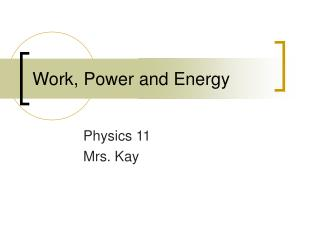Download PresentationWork, Power and Energy

# Work, Power and Energy

Download Presentation## Work, Power and Energy

- - - - - - - - - - - - - - - - - - - - - - - - - - - E N D - - - - - - - - - - - - - - - - - - - - - - - - - - -
##### Presentation Transcript

1. Work, Power and Energy Physics 11 Mrs. Kay

2. Work • The product of the force exerted on an object and the distance the object moves in the direction of the force. • W=FcosӨd • Units = J (joule)= N (newton) x m (metre) • Work is done only if an object moves

3. Force vs. Displacement • The area under a force displacement graph equals the work done.

4. If movement is in same direction • Holding objects doesn’t count as work, because no movement. • If perpendicular to movement cos(90o)=0, so no work. • If parallel to movement cos(Oo) =1, so W=Fd

5. Problem: • How much work is done by a weight lifter is she holds a 100N barbell above her head? Answer: W =FcosӨd =100N cos(90o)(Om) = 0 J

6. Problem 2: 2. A man carries a 50N box on his shoulder and walks 30m. How much work does he do on the box? Answer: W =FcosӨd =50N cos(90o) 30m =O J

7. Problem 3: • A girl exerts a force of 35N at a 35o angle to pull her wagon 25m down a street. How much work does she do on the wagon? Answer: W =FcosӨd =(35N)(cos35o)25m = 717J

8. Problem 4: • A superhero stops a runaway bus by exerting a force of 200N against the motion of the bus for 12m. How much work does he do on the bus? Answer: W =FcosӨd =(200N)(cos 180o) (12m) =-2400J (negative because in opposite direction of motion)

9. Remember: • If force and motion are horizontal and in the same direction you can use: • W=Fd

10. When No force is done

11. Work against gravity • When there is a vertical and horizontal component, the displacement is equal to the height or vertical component. It does not depend on the incline. • Ex: like a stairs or escalator question. • Use W=mgh, where h is the height that the object has traveled.

12. Problems: • A 75kg firefighter climbs up a flight of stairs 10m high. How much work is required? • A 900N crate rests on the floor. How much work is required to pull it 6m up a 35o incline? • A girl holds a 15kg bag above her head. How much work did it take for her to lift it up to 2.2m?

13. Answers: • W=mgh, (75kg)(9.8m/s2)(10m)=7350J • Vertical = sin(35) x 6 = 3.44m, so… W=Fd=(900N)(3.44m) =3097J • W=mgh = (15kg)(9.8m/s2)(2.2m)=323.4J

14. Practice: Day One: • Pg 199 #1-3 • Pg 202 # 5-7 • Pg 213 1-3 and 5 Day Two: • Pg 213 # 7-11

15. Power • The rate of doing work • P=W/t • Units: watt (w) = 1 Joule of energy transferred per second.

16. Problems: • Pg.203 #9-11 • Pg.214 # 12-13,15,18

17. Forms of Energy • Kinetic energy: found in moving objects • Potential energy: stored energy. It is converted into kinetic energy. Can have many forms within it. (elastic, gravitational, chemical…) • Energy is measured in Joules (J)

18. Kinetic Energy • The kinetic energy of an object is given by the equation: Ek=1/2 mv2 • It is proportional to the mass and square velocity of the object. • The heavier and more quickly the object is moving, the greater the kinetic energy

19. If there is a constant acceleration, and therefore a constant net force, and we assume the object is originally at rest, then W=Ek, or work is equal to kinetic energy

20. Work-Energy Theorem • Not all objects start at rest. • They may begin with work exerting on them, giving them an original Ek. • W-E Theorem states: The change in the kinetic energy of an object is equal to the net work done on it. Wnet = Ekf – Eki = Ek

21. W-E theorem • If net work is positive then Ek increases. Ex: Pitch a ball forward, the ball and force are in same direction so net work is positive.

22. If net work is negative then Ek decreases. Ex: catch the ball with mit, the balls motion if forward, but the mitt exerts a force opposite to the motion on the ball because the ball is slowed to zero Ek. The mitt exerts negative work on the ball.

23. Potential Energy • As you throw up a block, it loses kinetic energy (b/c it is slowing down), however it is gaining gravitational potential energy

24. Gravitational Potential Energy • Any time an object is thrown up, it must come down. Its downward motion is losing kinetic energy, but gaining potential energy • Ep=mgh (is only valid if g is constant) • Enet= Ek + Ep

25. Elastic Potential Energy • Energy can be stored in bending or stretching objects (like springs or elastics) • k= spring’s constant • x= distance spring is stretched

26. Practice: • Pg.221 #1-3 (Ek) • Pg.224 # 5-7 (Ep) • Pg. 237 #1-4 (Problems)